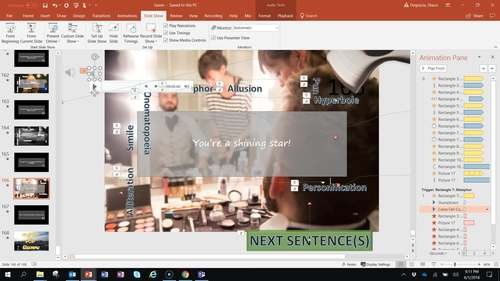# Math Facts & Vocabulary Interactive Game for PPTSubject
Resource Type
File Type
Presentation (Powerpoint) File (47 MB|613 pages)
Standards
\$4.99
• Product Description
• Standards
https://youtu.be/Rrj8oIiCAjE
This game is a PowerPoint file so it needs no special software. You can even hyperlink to it or copy and paste the slides into your presentations. It works best when you let the student hold the mouse or use the track pad. The e-beam is not as fun because the obstacles are no longer in effect without the cursor.
Model with mathematics. Mathematically proficient students can apply the mathematics they know to solve problems arising in everyday life, society, and the workplace. In early grades, this might be as simple as writing an addition equation to describe a situation. In middle grades, a student might apply proportional reasoning to plan a school event or analyze a problem in the community. By high school, a student might use geometry to solve a design problem or use a function to describe how one quantity of interest depends on another. Mathematically proficient students who can apply what they know are comfortable making assumptions and approximations to simplify a complicated situation, realizing that these may need revision later. They are able to identify important quantities in a practical situation and map their relationships using such tools as diagrams, two-way tables, graphs, flowcharts and formulas. They can analyze those relationships mathematically to draw conclusions. They routinely interpret their mathematical results in the context of the situation and reflect on whether the results make sense, possibly improving the model if it has not served its purpose.
Fluently multiply and divide within 100, using strategies such as the relationship between multiplication and division (e.g., knowing that 8 × 5 = 40, one knows 40 ÷ 5 = 8) or properties of operations. By the end of Grade 3, know from memory all products of two one-digit numbers.
Total Pages
613 pages